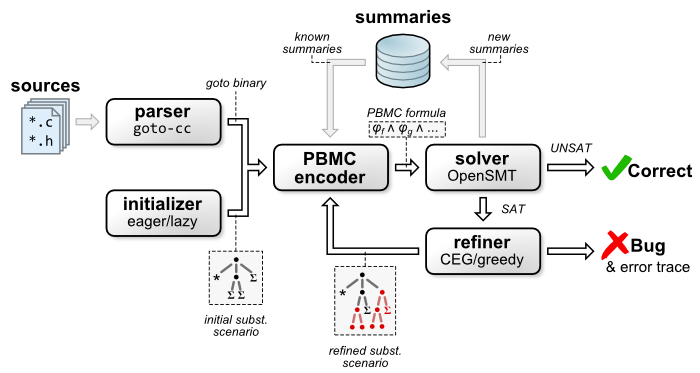# FunFrog architecture

Key features of the FunFrog are function summarization and refinement loop. The architecture is depicted in the following diagram:First, the source codes have to be parsed and a goto-binary is created. Then based on the chosen initial substitution scenario (eager - detailed processing of the functions without available summarieslazy - functions without summaries are treated as non-deterministic), the partitioned bounded model checking formula (PBMC formula) is constructed. This formula has two important properties (i) it is satisfiable if and only if an assertion violation is possible, and (ii) it has a form suitable for summary extraction using Craig interpolation (i.e., conjunction of parts representing individual functions).

The PBMC formula is passed to a solver (currently PeRIPLO) for a satisfiability check. If the formula is unsatisfiable then the assertions in the program hold and function summaries are generated using interpolation and stored to a permanent storage for future reuse.

If the PBMC formula is satisfiable then either a real or a spurious error is found. The refiner then attempts to identify function summaries directly involved in the error and marks the corresponding calls for precise analysis in the next iteration. If no such summaries are identified, the error is genuine and it is reported to a user.